# Moments of $S(T)$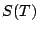is defined by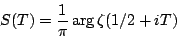where the argument is obtained by continuous variation from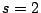where the argument is 0, to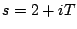to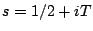, circumventing zeros of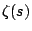by small semicircular detours above the zeros. Selberg [ MR 8,567e] proved an asymptotic formula for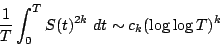for positive integral values of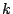and an appropriate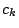. Goldston [ MR 89a:11086], assuming the Riemann Hypothesis, was able to give a second main term in the case that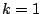. Keating and Snaith's conjectures for moments of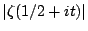imply formula for the above moments of, including lower order terms all the way to a constant, i.e. they conjecture that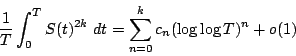for some explicit constants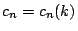.

It seems like further work should allow one to obtain the lower order terms in the moments of; it's possible that the assumption of the Riemann Hypothesis will allow for the evaluation of some of the lower order terms, and the assumption of GUE will allow for the rest.

Back to the main index for L-functions and Random Matrix Theory.